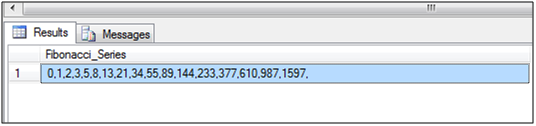# 1.     Background

The purpose of this article is to describe how to generate Fibonacci series in SQL Server. As per the definition of Fibonacci series, first two numbers are 0 and 1 and each subsequent number is the sum of previous two numbers in the series.

Fibonacci series: 0,1,2,3,5,8,13,21,34,55,89,144,233,377,610,987,1597.

Following are the places where we can utilize Fibonacci series:

·         MERGE sort
·         Search Algorithms
·         Network Topology
·         Lossy compression in Data encoding, Streaming Media, and internet telephony.

# 2. Script to generate Fibonacci Series in SQL Server: We are usingWHILE loop and COALESCE function for generating the Fibonacci series. After providing 0 and 1 as Initial values, we are generating series as per logic of Fibonacci series. COALESCE function is used to convert the rows data into comma separated Fibonacci Series. Below is the T-SQL script: /* Declaring variables */

DECLARE @F0 INT,
@F1 INT,
@LIMIT INT ,
@Fibonacci_Number NVARCHAR(MAX),
@Fibonacci_Series NVARCHAR(MAX)

/* Setting the inital value of Fibonacci Series */
SET  @F0 = 0
SET @F1 = 1

/* Set the limit for series*/
SET @LIMIT = 1000
/* Declaring table variable to save Fibonacci numbers as rows */
DECLARE @Fibonacci_Table TABLE
(ID INT)

/* Inserting Initial value 0 for Fibonanci Series*/
INSERT INTO @Fibonacci_Table
SELECT 0

/* Starting Loop to generate Fibonancci series*/
WHILE @LIMIT >= @F1
BEGIN
/* applying logic of Fibonancci series F= A+B */
SET @Fibonacci_Number = @F0 + @F1

/* Inserting the resultant number*/
INSERT INTO @Fibonacci_Table
SELECT @Fibonacci_Number

/*Assigning value A<=B */
SET @F0 = @F1
SET @F1 = @Fibonacci_Number
END
/* Converting Table's row into comma separated string */
SELECT @Fibonacci_Series = COALESCE (@Fibonacci_Series,'') + CAST (ID AS VARCHAR (50)) +','
FROM @Fibonacci_Table

/*Getting Fibonancci Series*/
SELECT @Fibonacci_Series As Fibonacci_Series

Output:# 3.     Conclusion

By using above steps, we can generate the Fibonacci Series in SQL Server.

1.thanks for logic

2.I sometimes like to discover new posts on blogs. Kim

3.Congratulations, excelent, perfect, good job.

4.This is just the information I am finding everywhere. Thanks for your blog, I just subscribe your blog. This is a nice blog.. Visit